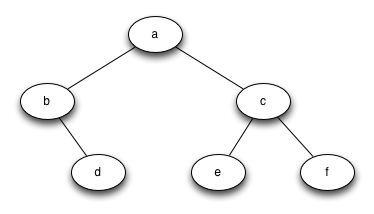TORONTO KIDS COMPUTER CLUB | PMCA Friday 19:00 Python Homework 20.02.28.
17147

# PMCA Friday 19:00 Python Homework 20.02.28.

## 03 Mar PMCA Friday 19:00 Python Homework 20.02.28.

Question 1:
Using the binaryTree() and other helper functions we made in the class. If we run the following code, please print the binary tree as list:

```r = binaryTree('a')
insertLeft(r,'b')
insertRight(r,'c')
insertRight(getRightChild(r),'d')
insertLeft(getRightChild(getRightChild(r)),'e')```

And also please draw the tree graph.

Question 2:
Write a function buildTree() that returns a tree using the list of lists functions that looks like this: